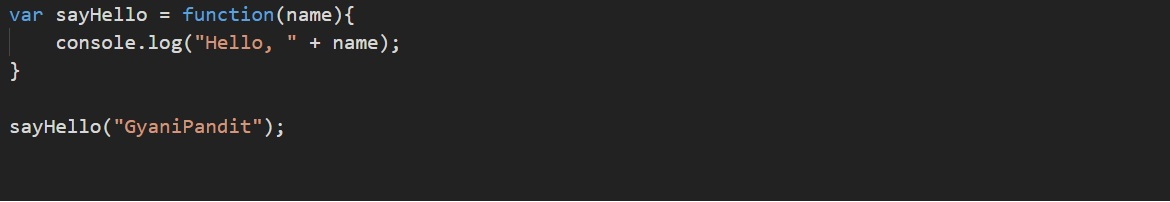# Function expression in Javascript

Function expression Javascript

Well, there is another way to create functions. The way we are going to discuss now, helps us create anonymous functions, which means that a function, that does not have a name.

However, You can also provide a name for the function with a function expression (don’t worry, we will get it with the example).

Let’s have a look at how we can create functions this way –As you can see, the syntax is pretty similar to how we used to do this before, but now, the difference is the function name. Here, the function name is not present, due to which, we can create an anonymous function. However, you can give a name to the function here as well.

Using the functions can be very handy, if we have some code, which we are going to use repeatedly, we just need to write it once into the function, and then we can use that code whenever we want. We just have to call the function. We might require to create functions to do something in our programs, and whenever we will require the functions, we are going to create them.